# Message Board

 Message Board > Malarkey > Mathematical frustrations <3

 April 26, 2013, 12:37 Zomg None 641 posts I would like to share with you all my mathematical frustrations. I spent 1 hour and thirty minutes racking my brain on equations, while making stupid mistakes such as distributing division with right hand left side terms (which is not allowed in math!) Behold: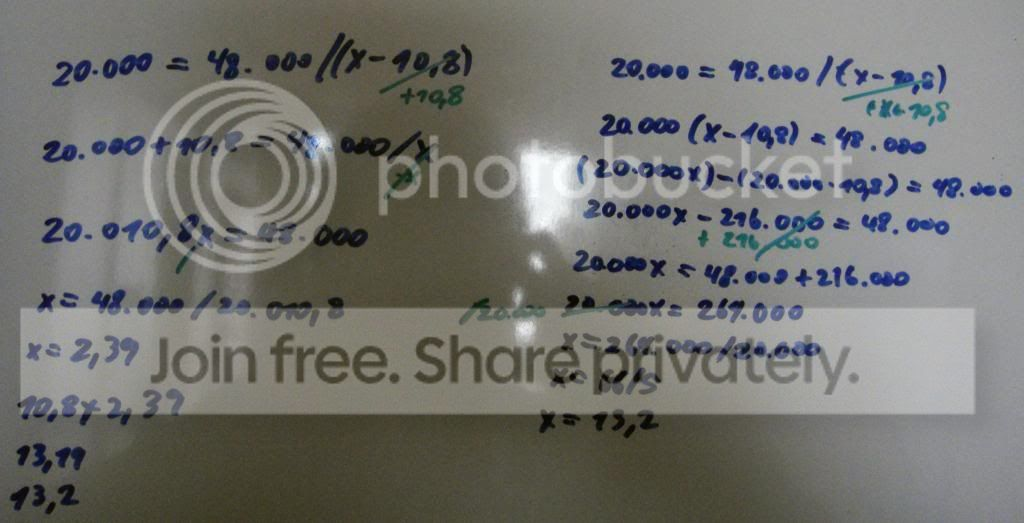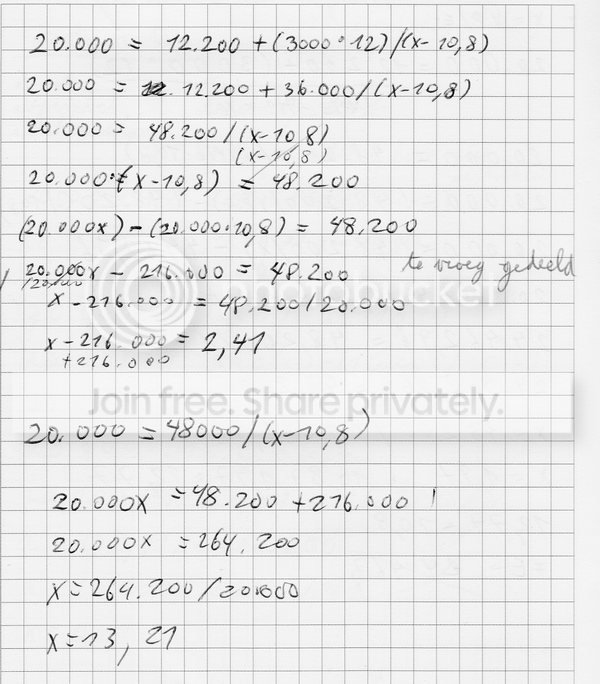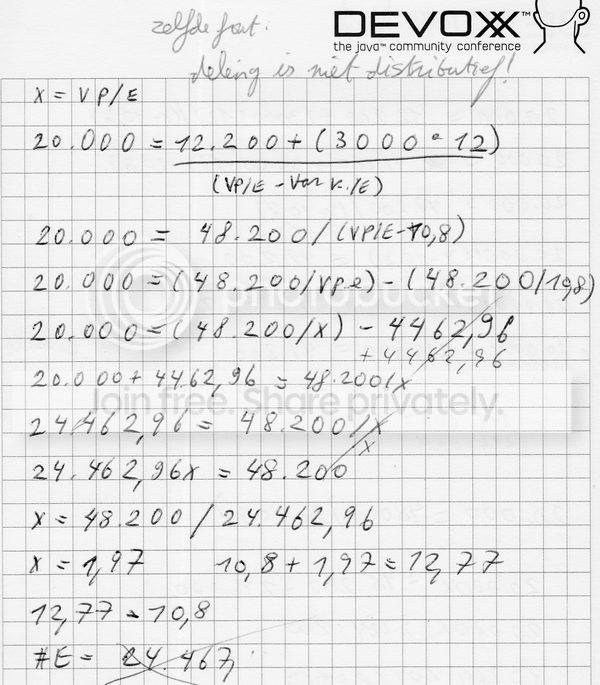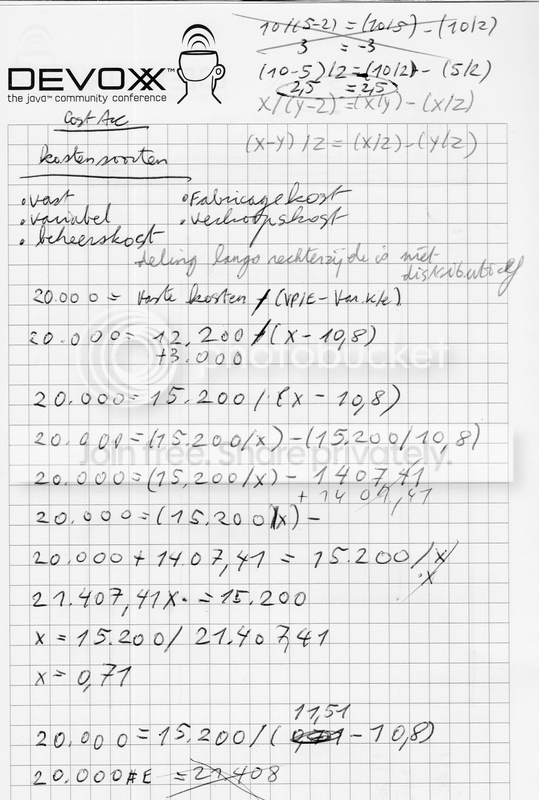Freaking math. Damn man. =P ____________ #
 April 29, 2013, 09:54 Dennis どこかにいる 2050 postsFind x,y: sqrt(7y + 10x) = x + y find x,y,z,a,b,c: sqrt(8y+12-27/z + (3c^3) + b) = ((12x-3z+a-(b^2))*(-3)-1)*-1[Edited on April 29, 2013 by Dennis] ____________ Kwakkel #
 April 29, 2013, 11:13 Dennis どこかにいる 2050 postsThe first one is x=6 and y=3, but you can also use x=0 and y=0 These are bad examples. Never mind.[Edited on April 29, 2013 by Dennis] ____________ Kwakkel #
 April 29, 2013, 14:43 Zomg None 641 posts =P. Yes, I do not know how to do two-term (discriminant), four-term (quadratic) or further (unchain in factorials) equations. I am still at equations with one unknown, which suffices for Kots Accounting. =D We still have to work with stuff like vierkantsvergelijking, if you are familiar with this. It's a little number in front of a sqrt() sign. Like: ³sqrt() ____________ #
 April 30, 2013, 09:41 Dennis どこかにいる 2050 postsI think you mean square root (vierkantswortel) cube root (derdemachtswortel) and n root (dunno the dutch term) a square root, is finding the factor that multiplies itself vierkantsvergelijking is an quadratic equation like a² + bx + c = 0 SQRT tutorial sqrt sqrt sqrt! Just kidding. Real SQRT tutorial sqrt(25) = 5, because 5² = 25. Finding a square root is done by guessing, learning by heart, or using a calculator. There IS a method to do it with pencil and paper but those still require some guessing, so it's best to use a calculator. This is the order: ALWAYS: Between brackets from deepest level and up FIRST! Locate the brackets and solve them first. 1. powers and roots: roots: do what's under the upper line of √ first! 2. multiply and division: left to right 3. adding and substraction: left to right example: __________ ³√8-1+2²*5 + 4 -(8+3*(7+3)) Locate deepest brackets and solve what's between them so you can remove the brackets: __________ ³√8-1+2²*5 + 4 -(8+3*(7+3)) It's solved so we can go a level higher: __________ ³√8-1+2²*5 + 4 -(8+3*10) Follow the order above. 1: powers and roots -> none 2: multiplying and division: __________ ³√8-1+2²*5 + 4 -(8+3*10) __________ ³√8-1+2²*5 + 4 -(8+30) 3: adding and substraction: __________ ³√8-1+2²*5 + 4 -(38) Remove the brackets if there are no more operations: __________ ³√8-1+2²*5 + 4 -38 There are no more brackets, but what's under the root's upper line is considered "between brackets": ___________ ³√8-1+2²*5 + 4 -38 1: powers and roots: ___________ ³√8-1+4*5 + 4 -38 2: multiplying and division: __________ ³√8-1+20 + 4 -38 3: adding and substraction (from LEFT to RIGHT) _________ ³√8-1+20 + 4 -38 _______ ³√7+20 + 4 -38 ____ ³√27 + 4 -38 No more operations below the root's upper line! No more brackets to be found nowhere! 1: powers and roots: ____ ³√27 + 4 -38 Guess: ____ ³√27 = x do this operation on both sides: ^3 27 = x³ <=> 27 = x*x*x <=> 27 = 3*3*3 // so the result is 3. Use a calculator to omit that shit and see "3" on your screen. 2: Multiplying and division: none 3: adding and substraction: (from LEFT to RIGHT) 3 + 4 - 38 7 - 38 eventual result: -31 by the way: note that a SQUARE root can have 2 results: x and -x. 5*5 = 25 -5*-5 = 25 sqrt(-25) or sqrt(-16) results to the imaginary number i, because there is no real number that can result to a negative result squared. See the result as a variable. So mind that (-x)*(-x) is always positive, (You can clearly see why if you draw it on a graph) except i*i. That's why sqrt(25) can be either 5 or (-5). The result of x² is always positive, but the special imaginary number i results to -x when squared. We actually agree to it that there EXISTS a special number that we cannot represent visually that CAN result into a negative number squared. This may look weird at first, but it is not weirder than Romans who found it weird to represent 0 as a number. They were like: "why use a number for something that doesn't EXIST? Why use "nothing" in a calculation? You cannot sell 0 breads, it's pointless!" Well this is the same case with i. Also: follow reason is why 0 doesn't exist in roman numerals!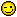Bien à toi Kind regards Vriendelijke groeten あなたに感謝し、敬具 ____________ Kwakkel #
 April 30, 2013, 11:43 Zomg None 641 posts Thank you ^1000000000, Dennis. You are a nice and patient person. =) I always wondered in French what 'BoT' meant, by I quickly figured out it was indeed 'Bien à toi'. =P ____________ #

Message Board > Malarkey > Mathematical frustrations <3